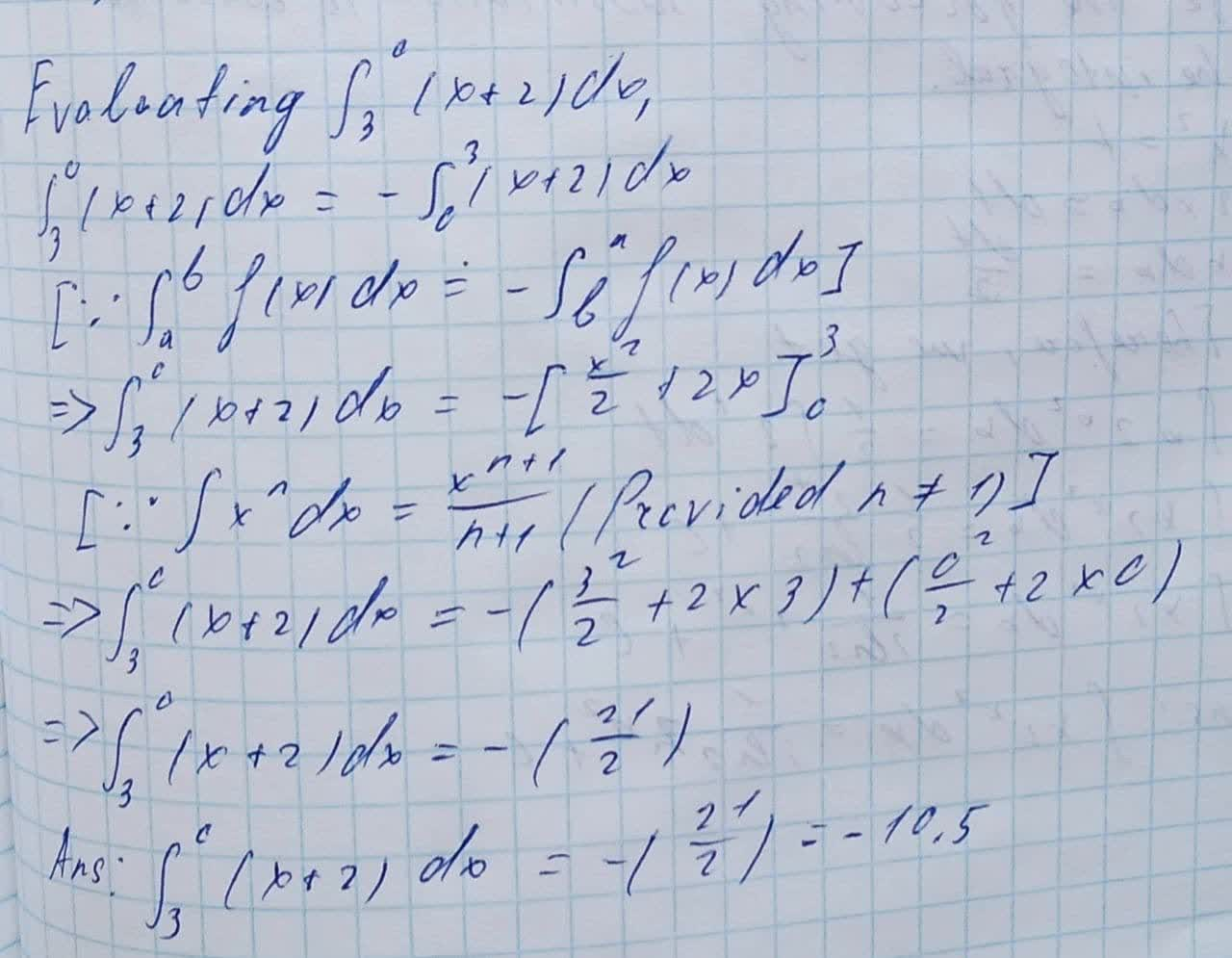Question# Evaluate the definite integral: \int_{3}^{0} (x+2)dx

Applications of integrals
ANSWEREDEvaluate the definite integral.
$$\displaystyle{\int_{{{3}}}^{{{0}}}}{\left({x}+{2}\right)}{\left.{d}{x}\right.}$$